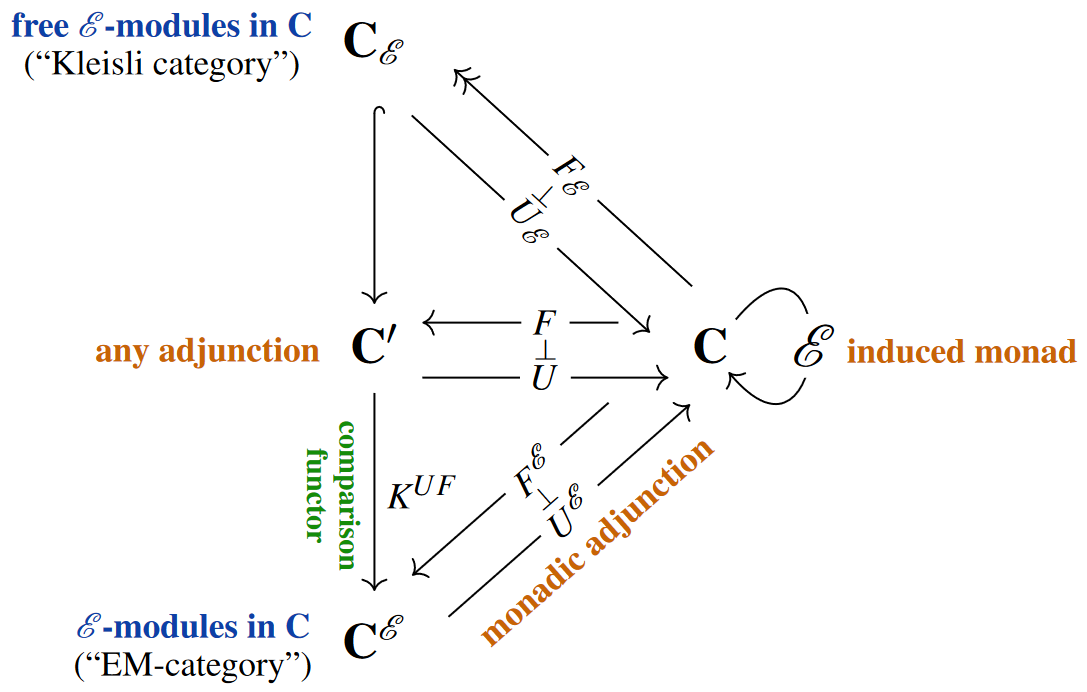# nLab comparison functor

Contents

### Context

category theory

#### Categorical algebra

internalization and categorical algebra

universal algebra

categorical semantics

# Contents

## Idea

While every functor might be understood as constituting a “comparison” between its domain and codomain-categories, the term “comparison functor” is often understood by default as referring, in categorical algebra, to the unique functor that relates any adjunction to its monadic adjunction – this is the case we discuss here.

But other instances of functorial “comparison” are bound to be relevant. For instance, for the “comparison lemma” in topos theory see there.

## Statement

###### Proposition

Every pair of adjoint functors $(F \dashv U) \;\colon\; \mathbf{C}' \leftrightarrow \mathbf{C}$ (with unit $\eta^{U F}$ and counit $\epsilon^{F U}$) between categories $\mathbf{C}$, $\mathbf{C}'$ fits into a commuting diagram in Cat of the following form:Here:

• $\mathcal{E} \;\coloneqq\; U F \;\coloneqq\; U \circ F$ denotes the induced endofunctor on $\mathbf{C}$

which carries the structure of a monad with

• unit given by

$\eta^{\mathcal{E}} \;\; \colon \;\; id_{\mathbf{C}} \xrightarrow{\;\; \eta^{U F} \;\;} U F \;=\; \mathcal{E}$
• product given by

$\mu^{\mathcal{E}} \;\; \colon \;\; \mathcal{E} \mathcal{E} \;=\; U F U F \xrightarrow{\;\; U ( \epsilon^{F U} ) F \;\;} U F \;=\; \mathcal{E}$
• $\mathbf{C}^{\mathcal{E}}$ denotes the (“Eilenberg-Moore”-)category of $\mathcal{E}$-algebras in $\mathbf{C}$

$A \;=\; \Big( \mathcal{E} U^{\mathcal{E}}(A) \xrightarrow{\;\; \rho_A \;\;} U^{\mathcal{E}}(A) \Big)$

in $\mathbf{C}$ with homomorphisms between them,

• $K^{U F}$ denotes the “comparison functor” given by

$\array{ \mathbf{C}' & \xrightarrow{\;\;\;\;\;\;\;\;\;\; K^{U F} \;\;\;\;\;\;\;\;\;\;} & \!\!\!\!\!\!\!\!\!\!\!\!\!\! \mathbf{C}^{\mathcal{E}} \\ C' &\mapsto& { \Big( \mathcal{E} U(C') \,=\, U F U (C') \xrightarrow{\; U \epsilon^{F U}_{C'} \;} U (C') \Big) } }$
• $\mathbf{C}_{\mathcal{E}}$ denotes the (“Kleisli”-)category of free $\mathcal{E}$-algebras

$\begin{array}{rl} F^{\mathcal{E}}(C) & \;=\; \Big( \mathcal{E} \mathcal{E} (C) \xrightarrow{\;\; \mu^{\mathcal{E}}_C \;\;} \mathcal{E}(C) \Big) \\ \;=\; K^{U F} F(C) & \;=\; \Big( \mathcal{E} U F (C) \xrightarrow{\;\; U \epsilon^{ F U }_{F(C)} \;\;} U F (C) \Big) \,. \end{array}$
• Here the last line makes explicit that

$U^{\mathcal{E}} F^{\mathcal{E}} \;=\; U F \;=\; \mathcal{E} \,.$

In fact, $F^{\mathcal{E}} \dashv U^{\mathcal{E}}$ and this realizes the monadic adjunction whose induced monad is $\mathcal{E}$.

Hence the original $F \dashv U$ is monadic iff the comparison functor $K^{U F}$ is an equivalence of categories.

(See for instance MacLane (1971), §VI.3)

## Properties

The initiality of the Kleisli category under monad morphisms was first observed in

Textbook account: